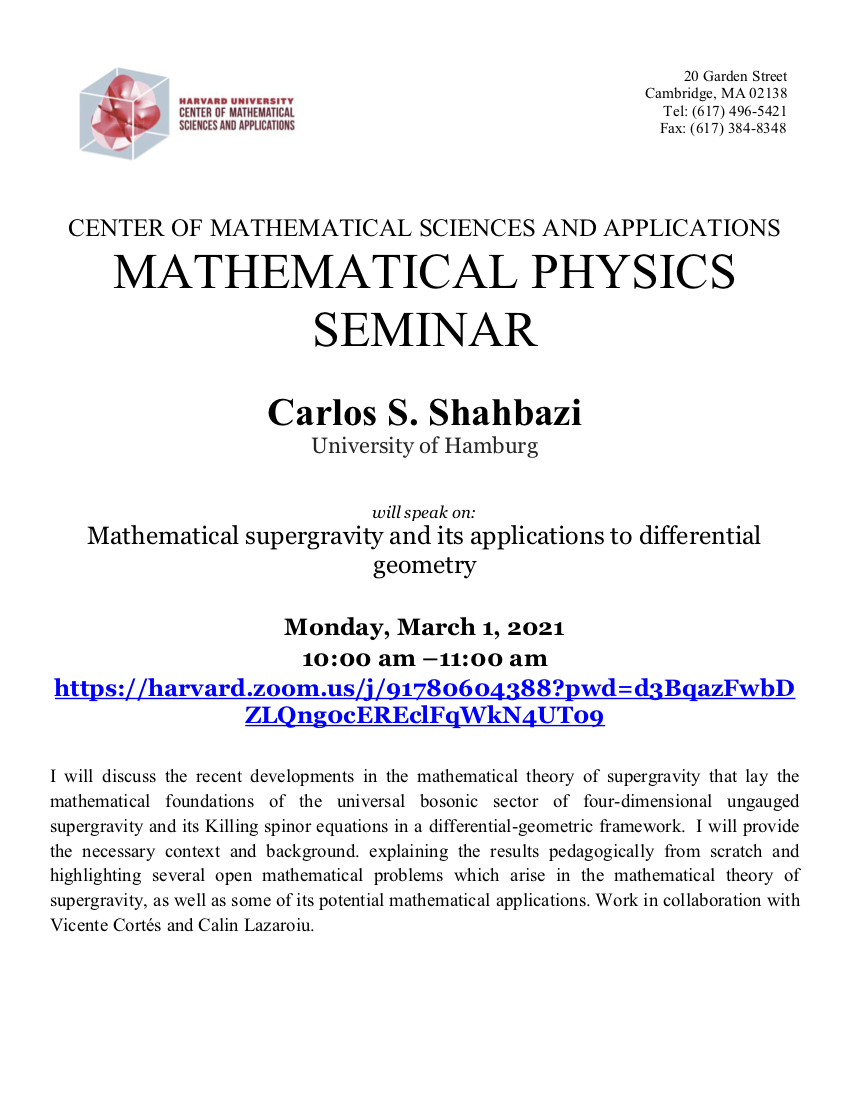# Mathematical supergravity and its applications to differential geometry

03/01/2021 10:00 am - 11:00 am
Address: Virtual and in 20 Garden Street, Room G10Speaker: Carlos S. Shahbazi (Hamburg University)

Title: Mathematical supergravity and its applications to differential geometry

Abstract: I will discuss the recent developments in the mathematical theory of supergravity that lay the mathematical foundations of the universal bosonic sector of four-dimensional ungauged supergravity and its Killing spinor equations in a differential-geometric framework.  I will provide the necessary context and background. explaining the results pedagogically from scratch and highlighting several open mathematical problems which arise in the mathematical theory of supergravity, as well as some of its potential mathematical applications. Work in collaboration with Vicente Cortés and Calin Lazaroiu.